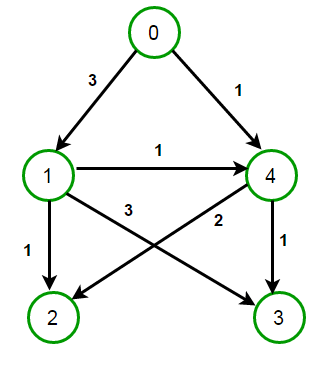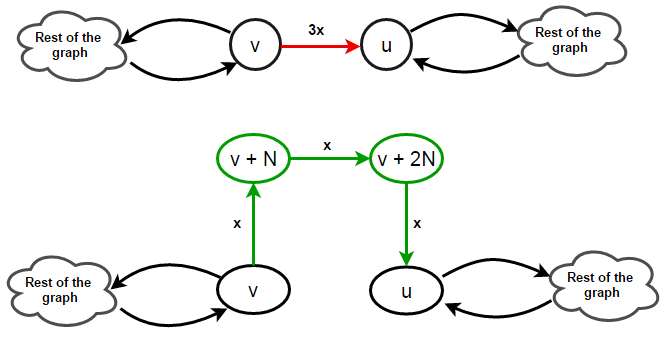# Least Cost Path in Weighted Digraph using BFS

Consider a directed graph where weight of its edges can be one of x, 2x or 3x (x is a given integer), compute the least cost path from source to destination efficiently.

For example, consider below graphIf source is 1 and destination is 3,
least cost path from source to destination is [1, 4, 3] having cost 2.

If source is 0 and destination is 2,
least cost path from source to destination is [0, 4, 2] having cost 3.

We know that breadth-first search can be used to find shortest path in an unweighted graph or in weighted graph having same cost of all its edges. BFS runs in O(E+V) time where E is the number of edges and V is number of vertices in the graph. But if the edges in the graph are weighted with different costs, then the recommended algorithm is Dijkstra’s Algorithm which takes O(E log V) time.

#### Can we use BFS?

The idea is to modify the input graph in such a way that all its edges have same weight. For edges having weight 3x, we split them into three edges of weight x each. Similarly, edges having weight 2x gets split into two edges of weight x each. Nothing needs to be done for edges already having weight x. Special care has to be taken while introducing new edges in the graph such that we should not introduce new routes into the graph. So in order to split an edge of weight 3x, we need to create two new vertices in the graph instead of using existing vertices. Similarly, in order to split edge having weight 2x, we need to create one new vertex. Lets illustrate this with the help of a diagram.

Split edge (v, u) having weight 3x into three edges (v, v+N), (v+N, v+2N) and (v+2N, u) each having weight x.Split edge (v, u) having weight 2x into two edges (v, v+N) and (v+N, u) each having weight x.## C++

Output:

Least cost path between 0 and 2 is 0 4 2 having cost 3

## Java

Output:

Least cost path between 0 and 2 is 0 4 2 having cost 3(1 votes, average: 5.00 out of 5)Loading...

Please use our online compiler to post code in comments. To contribute, get in touch with us.
Like us? Please spread the word and help us grow. Happy coding 🙂Subscribe
Notify ofGuest

very nice.. thanks!Guest
eromeroc97

what is N in the BFS(Graph graph, int source, int destination, int N) method